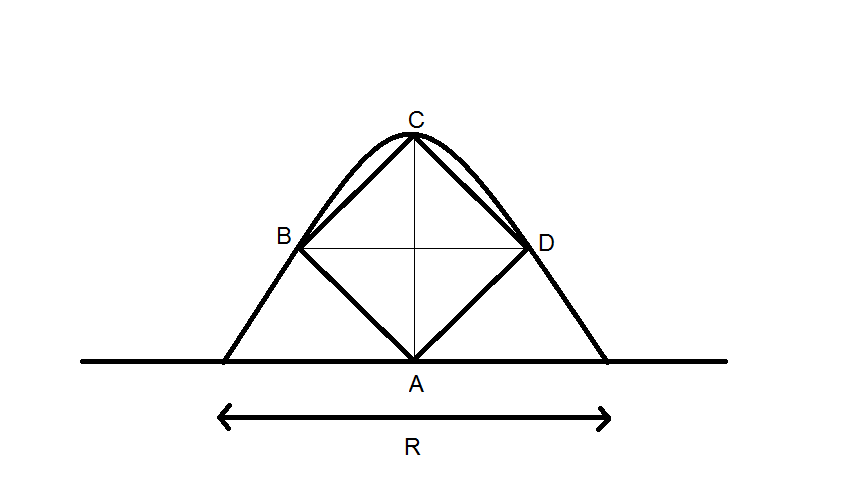# Projectile over a Square (part -2)A Square rests on a horizontal plane in such a way that the diagonals of the square are vertical and horizontal. A projectile is thrown from level ground in such a way that the projectile touches all the three vertices which lie in air. If side length of square is $20$ metres then find the angle of projection.
Note: The angle of projection is of the form $\theta = tan^{-1}a\sqrt{b}$

Find $a + b$

×